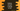# Python program to convert a string to list## Python program to convert a string to a list:

Converting a string to a list is not tough. In this post, we will learn how to convert a string to list of words or list of characters.

In this post, we will learn how to implement these in python with examples.

### Convert a string to list of characters in python:

Let’s convert a string to a list of characters first. This is actually simple. We can use list() method and pass the string as argument to this method to convert that string to a list of characters.

Let’s take a look at the below code:

``````given_string = 'hello'
given_string_second = 'hello world !!'

char_list = list(given_string)
char_list_second = list(given_string_second)

print(char_list)
print(char_list_second)``````

It will print the below output:

``````['h', 'e', 'l', 'l', 'o']
['h', 'e', 'l', 'l', 'o', ' ', 'w', 'o', 'r', 'l', 'd', ' ', '!', '!']``````

So, list will include all characters to a list including the spaces.

### String to a list of strings:

We can also use the split method to convert a string to a list of strings. For example, we can split a string with any separator. We can optionally pass a separator to split or it will take the whitespace as its separator.

For example, take a look at the below example:

``````given_string = 'hello world !!'
given_string_second = 'one,two,three,four,five'

first_list = given_string.split()
second_list = given_string_second.split()

print(first_list)
print(second_list)``````

It will print the below output:

``````['hello', 'world', '!!']
['one,two,three,four,five']``````

### String of integers to list of integers:

We can also convert a string that holds only integers to a list of integers.

``````given_string = '1,2,3,4,5,6,7,8,9'

char_list = given_string.split(',')
print(char_list)

int_list = [int(c) for c in char_list]
print(int_list)``````

Here,

• split is used to convert the string to a list of characters.
• Using a for loop again, we are converting the characters to integers.

It will print the below output:

``````['1', '2', '3', '4', '5', '6', '7', '8', '9']
[1, 2, 3, 4, 5, 6, 7, 8, 9]``````